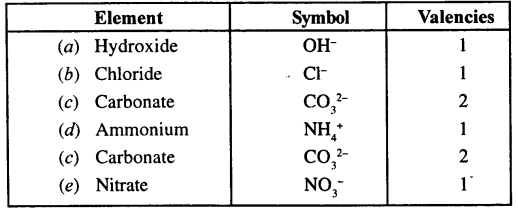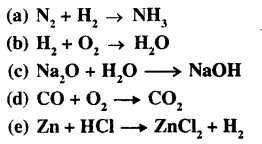## Selina Concise Chemistry Class 8 ICSE Solutions – Language of Chemistry

ICSESolutions.com provides step by step solutions for Selina Concise ICSE Solutions for Class 8 Chemistry Chapter 5 Language of Chemistry. You can download the Selina Concise Chemistry ICSE Solutions for Class 8 with Free PDF download option. Selina Publishers Concise Chemistry for Class 8 ICSE Solutions all questions are solved and explained by expert teachers as per ICSE board guidelines.

Selina Concise ICSE Solutions for Class 8 Chemistry Chapter 5 Language of Chemistry

Points to Remember:

• The valency of an element is the number of electrons donated or accepted by its ‘atom’ during chemical combination.
• There are some elements with more than one valency e.g., iron, copper, tin, lead.
• Two or more different non-metals that collectively accept or donate one or more electrons and become negatively or positively charged in the process are called radicals.
• A chemical reaction involves the transformation of original substance into an altogether new substance(s).
• A chemical reaction can be represented with the help of the symbols or the formulae of the elements and the compounds taking part in that reaction. This gives a chemical equation.
• Certain necessary conditions for a chemical reaction to happen are — close contact, solution form, heat, light and catalyst.
• Characteristics of chemical reactions are — change of colour, evolution of a gas, formation of a precipitate, change of state, change of smell and evolution/absorption of heat.
• A complete chemical equation symbolically represents the reactants, products and their physical states.
• The substances that react with each other are called reactants and they are represented on the left hand side of the equation. The substances that are formed as a result of the reaction are called products. They are represented on the right hand side of the equation.
• A chemical equation needs to be balanced to make it follow the law of the conservation of mass.
• The law of conservation of mass states that mass can be neither created nor destroyed, it can only be transformed from one form to another.
• A chemical equation gives both qualitative and quantitative information about the reactants and products.

ACTIVITY 1
Write the names and symbols of the first twenty elements that you have studied in class VI & VII.ACTIVITY 2
Write the molecular formulae of:

1. Copper oxide
2. Iron (III) chloride
3. Sodium hydroxide
4. Iron (II) sulphide
6. Hydrogen nitrate (nitric acid)
7. Hydrogen sulphate (sulphuric acid)
8. Calcium hydroxide
9. Magnesium carbonate
10. Ammonium carbonate

1. Copper oxide – CuO
2. Iron (III) chloride – FeCl3
3. Sodium hydroxide – NaOH
4. Iron (II) sulphide – FeS
5. Lead (II) oxide – PbO
6. Hydrogen nitrate (nitric acid) – HNO3
7. Hydrogen sulphate (sulphuric acid) – H2SO4
8. Calcium hydroxide – Ca(OH)2
9. Magnesium carbonate – MgCO3
10. Ammonium carbonate – (NH4)2CO3

ACTIVITY 3
Write the molecular formula for each of the following compounds:

1. Sulphur trioxide
2. Iron (II) sulphide and
3. Ammonia

Find the number and names of elements present in them and calculate their molecular masses.
1. Sulphur trioxide

1. A molecule of sulphur trioxide is represented by the formula SO3.
2. The elements present in it are sulphur dioxide and oxygen.
3. One molecule of sulphur trioxide has one atom of sulphur and three atoms of oxygen.
4. Molecular mass of sulphur trioxide (SO3)
= 32 + 3 x 16
= 32 + 48 = 80 amu.

2. Iron (II) sulphide

1. A molecule of iron (II) sulphide is represented by the formula FeS.
2. The elements present in it are iron and sulphur.
3. One molecule of iron (II) sulphide has one atom of iron and one atom of sulphur.
4. Molecular mass of iron (II) sulphide (FeS)
= 55.5 + 32
= 87.5 amu.

3. Ammonia

1. A molecule of ammonia is represented by the formula NH3.
2. The elements present in it are nitrogen and hydrogen.
3. One molecule of ammonia has one atom of nitrogen and three atoms of hydrogen.
4. Molecular mass of ammonia (NH3)
= 14 + 3 x 1
= 14 + 3
= 17 amu.

Exercise

Question 1.
Define:
(b) Valency
(c) Molecular formula
(a) Radical: A radical is an atom of an element or a group of atoms of different elements that behaves as a single unit with a positive or negative charge on it.
(b) Valency: It is the number of electrons donated or accepted by the valence shell of an atom during chemical combination.
(c) Molecular formula: It is a symbolic representation of a molecule. It shows the number of atoms of each element present in it. These atoms combine in the whole numbers to form the molecule.

Question 2.
Give the symbols and valencies of the following radicals:
(a) Hydroxide (b) Chloride
(c) Carbonate (d) ammonium
(e) NitrateQuestion 3.
Write the molecular formula for the oxide and sulphide of the following elements.
(a) Sodium (b) Calcium
(c) Hydrogen
(a) Sodium oxide Na2O
Sodium sulphide Na2S
(b) Calcium oxide CaO
Calcium sulphide CaS
(c) Hydrogen oxide H2O
Hydrogen sulphide H2S

Question 4.
Write the molecular formulae for the following compounds and name the elements present.
(a) Baking soda (b) Common salt
(c) Sulphuric acid (d) Nitric acid
(a) Baking soda — NaHCO3
Elements present in Baking soda are sodium, hydrogen, oxygen and carbon.
(b) Common salt — NaCl
Element present are: Sodium and chlorine.
(c) Sulphuric acid — H2SO4
Element present are: Hydrogen, sulphur and oxygen.
(d) Nitric acid — HNO3
Elements present are: Hydrogen, nitrogen and oxygen.

Question 5.
The valency of aluminium is 3. Write the valency of other radicals present in the following compounds.
(a) Aluminium chloride
(b) Aluminium oxide
(c) Aluminium nitride
(d) Aluminium sulphate
(a) Aluminium chloride — (AlCl3) here valency of Al is 3.
Valency of chloride = 1
(b) Aluminium oxide — (Al2O3)
Here valency of Al is 3
Other radical presents = oxide (O2-)
Valency of O2- = 2
(c) Aluminium nitride — (Al N)
Here valency of aluminium = 3
Valency of nitride (N3-) = 3
(d) Aluminium sulphate — Al2(SO4)3
Here valency of aluminium is 3
Valency of (SO42-) = 2

Question 6.
What is variable valency? Give two examples of elements showing variable valency.
Certain elements exhibit more than one valency, which means they show variable valency.
Ferrous is written as Iron (II) and Ferric is written as Iron (III).

 Metal Radicals Valency Iron Ferrous [Iron (II)] Ferric [Iron (III)] 2 3 Copper Cuprous [Copper (I)] Cupric [Copper (II)] 1 2

Question 7.
(a) What is a chemical equation?
(b) Why it is necessary to balance a chemical equation?
(c) What are the limitations of a chemical equation?
(a) Chemical Equation— A chemical equation is the symbolic representation of a chemical reaction using the symbols and the formulae of the substances involved in the reaction.
(b) A chemical equation needs to be balanced so as to make the number of the atoms of the reactants equal to the number of the atoms of the products.
(c)

1. It does not inform about the physical states of the reactants and the product i.e. whether they are solids, liquids, and gases.
2. It does not inform about the concentration of reactants and products.
3. It does not inform about the time taken for the completion of the reaction.
4. It does not inform about the rate at which a reaction proceeds.
5. It does not inform about the heat changes during the reaction i. e. whether the heat is given out or absorbed.
6. It does not inform about the conditions such as temperature, pressure, catalyst, etc. which affect the reaction.
7. It does not inform about the nature of the reaction i.e. whether it is reversible or irreversible.

Question 8.

1. The physical state of the reactants and products can be indicated by putting (s) for solid, (l) for liquid, (g) for gas and (aq) for aqueous state.
2. Evolution or absorption of heat during the reaction can be denoted by adding or subtracting a heat term on the product side.
3. Temperature, pressure and catalyst can be indicated above the arrow (→ or =) separating the reactants and products.
4. Concentration of reactants and products are indicated by adding word (dil) for dilute and (cone) for concentrated before their formulae.
5. By the sign → or $$\rightleftharpoons$$ information about irreversible and reversible reactions can be obtained.

Question 9.
State the law of conservation of mass.
Law of conservation of mass: It states that mass can neither be created nor destroyed in a chemical reaction. During any change (physical or chemical), matter is neither created nor destroyed. However it may change from one form to another.Experimental Verification of Law of Conservation of Mass

Requirements: H-shaped tube called Landolt’s tube, Sodium chloride solution, silver nitrate solution, etc.
Procedure: A specially designed H-shaped tube is taken. Sodium chloride solution is taken in one limb of the tube and silver nitrate solution in the other limb as shown in the figure.
Both the limbs are now sealed and weighed. Now the tubes is averted so that the solutions can mix up together and react chemically. The reaction takes place and a white precipitate of silver chloride is obtained.The tube is weighed again. The mass of the tube is found to be exactly the same as the mass obtained before inverting the tube.
Thus, this experiment clearly verifies the law of conservation of mass.

Question 10.
Differentiate between:
(a) Reactants and products
(b) A balanced and an unbalanced chemical equation
(a) Reactants and products

Reactants

1. The substances that react with one another are called reactants.
2. Reactants are written on the left-hand side of the equation.

Products

1. The new substances formed are called products.
2. Products are written on the right-hand side of the equation.

(b) A balanced and an unbalanced chemical equation

Balanced chemical

1. A balanced chemical reaction is one in which the number of atoms of each element on the reactant side is equal to the number of atoms of that element on the product side.
2. Ex- H2 + Cl2 → HCl

Unbalanced chemical

1. The number of elements on the reactant side is not equal to the number of elements on the product side.
2. Ex- H2 + Cl2 → 2HCl

Question 11.
Balance the following equations:Question 12.
12. Write balanced chemical equations for the following word equations:
(a) Iron + Chlorine → Iron (III) chloride
(b) Magnesium + dil sulphuric acid → Magnesium sulphate + water
(c) Magnesium + oxygen → Magnesium oxide
(d) Calcium oxide + water → Calcium hydroxide
(e) Sodium + chlorine → Sodium chloride
(a) Iron + Chlorine → Iron (III) chloride
4Fe + 3Cl2 → 2F2Cl3
(b) Magnesium + dil sulphuric acid → Magnesium sulphate + water
2Mg + 2H2SO4 → 2MgSO4 + 2H2
(c) Magnesium + oxygen → Magnesium oxide
2Mg + O2 → 2MgO
(d) Calcium oxide + water → Calcium hydroxide
CaO + H2O → Ca(OH)2
(e) Sodium + chlorine → Sodium chloride
2Na + Cl2 → 2NaCl

Question 13.
What information do you get from the following chemical equation:
Zn(s) + 2HCl (dil) → ZnCl2 (aq) + H2(g)